Most Affordable JEE | NEET | 8,9,10 Preparation by Kota's Top IITian Doctor Faculties

# NCERT Solutions for Class 12 Chemistry Chapter 2 Solutions PDF - eSaral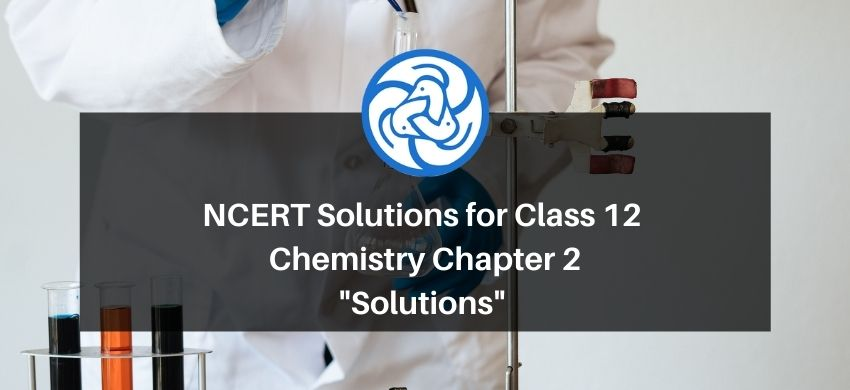`

# NCERT Solutions for Class 12 Chemistry Chapter 2 Solutions PDF

Hey, are you a class 12 student and looking for ways to download NCERT Solutions for Class 12 Chemistry Chapter 2 Solutions PDF? If yes. Then read this post till the end.

In this article, we have listed NCERT Solutions for Class 12 Chemistry Chapter 2 Solutions in PDF that are prepared by Kota’s top IITian Faculties by keeping Simplicity in mind.

If you want to learn and understand class 12 Chemistry Chapter 2 "Solutions" in an easy way then you can use these solutions PDF.

NCERT Solutions helps students to Practice important concepts of subjects easily. Class 12 Chemistry solutions provide detailed explanations of all the NCERT questions that students can use to clear their doubts instantly.

If you want to score high in your class 12 Chemistry Exam then it is very important for you to have a good knowledge of all the important topics, so to learn and practice those topics you can use eSaral NCERT Solutions.

So, without wasting more time Let’s start.

### Download NCERT Solutions for Class 12 Chemistry Chapter 2 Solutions PDF

Question 1: Calculate the mass percentage of benzene $\left(\mathrm{C}_{6} \mathrm{H}_{6}\right)$ and carbon tetrachloride $\left(\mathrm{CCl}_{4}\right)$ if $22 \mathrm{~g}$ of benzene is dissolved in $122 \mathrm{~g}$ of carbon tetrachloride.

Solution. Mass percentage of $\mathrm{C}_{6} \mathrm{H}_{6}=\frac{\text { Mass of } \mathrm{C}_{6} \mathrm{H}_{6}}{\text { Total mass of the solution }} \times 100 \%$

$=\frac{\text { Mass of } \mathrm{C}_{6} \mathrm{H}_{6}}{\text { Mass of } \mathrm{C}_{6} \mathrm{H}_{6}+\mathrm{Mass} \text { of } \mathrm{CCl}_{4}} \times 100 \%$

$=\frac{22}{22+122} \times 100 \%$

$=15.28 \%$

Mass percentage of $\mathrm{CCl}_{4}=\frac{\text { Mass of } \mathrm{CCl}_{4}}{\text { Total mass of the solution }} \times 100 \%$

$=\frac{\text { Mass of } \mathrm{CCl}_{4}}{\text { Mass of } \mathrm{C}_{6} \mathrm{H}_{6}+\mathrm{Mass} \text { of } \mathrm{CCl}_{4}} \times 100 \%$

$=\frac{122}{22+122} \times 100 \%$

$=84.72 \%$

Alternatively,

Mass percentage of $\mathrm{CCl}_{4}=(100-15.28) \%$

$=84.72 \%$

Question 2: Calculate the mole fraction of benzene in solution containing 30% by mass in carbon tetrachloride.

Solution. Let the total mass of the solution be $100 \mathrm{~g}$ and the mass of benzene be $30 \mathrm{~g}$.

$\therefore$ Mass of carbon tetrachloride $=(100-30) \mathrm{g}$

$=70 \mathrm{~g}$

Molar mass of benzene $\left(\mathrm{C}_{6} \mathrm{H}_{6}\right)=(6 \times 12+6 \times 1) \mathrm{g} \mathrm{mol}^{-1}$

$=78 \mathrm{~g} \mathrm{~mol}^{-1}$

$\therefore$ Number of moles of $\mathrm{C}_{6} \mathrm{H}_{6}=\frac{30}{78} \mathrm{~mol}$

$=0.3846 \mathrm{~mol}$

Molar mass of carbon tetrachloride $\left(\mathrm{CCl}_{4}\right)=1 \times 12+4 \times 35.5$

$=154 \mathrm{~g} \mathrm{~mol}^{-1}$

$\therefore$ Number of moles of $\mathrm{CCl}_{4}=\frac{70}{154} \mathrm{~mol}$

$=0.4545 \mathrm{~mol}$

Thus, the mole fraction of $\mathrm{C}_{6} \mathrm{H}_{6}$ is given as:

$\frac{\text { Number of moles of } \mathrm{C}_{6} \mathrm{H}_{6}}{\text { Number of moles of } \mathrm{C}_{6} \mathrm{H}_{6}+\text { Number of moles of } \mathrm{CCl}_{4}}$

$=\frac{0.3846}{0.3846+0.4545}$

$=0.458$

Question 3: Calculate the molarity of each of the following solutions: (a) $30 \mathrm{~g}$ of $\mathrm{Co}\left(\mathrm{NO}_{3}\right)_{2} .6 \mathrm{H}_{2} \mathrm{O}$ in $4.3 \mathrm{~L}$ of solution (b) $30 \mathrm{~mL}$ of $0.5 \mathrm{M} \mathrm{H}_{2} \mathrm{SO}_{4}$ diluted to $500 \mathrm{~mL}$.

Solution. Molarity is given by:

Molarity $=\frac{\text { Moles of solute }}{\text { Volume of solution in litre }}$

(a) Molar mass of $\mathrm{Co}\left(\mathrm{NO}_{3}\right)_{2} \cdot 6 \mathrm{H}_{2} \mathrm{O}=59+2(14+3 \times 16)+6 \times 18$

$=0.103 \mathrm{~mol}$

Therefore, molarity $=\frac{0.103 \mathrm{~mol}}{4.3 \mathrm{~L}}$

$=0.023 \mathrm{M}$

(b) Number of moles present in $1000 \mathrm{~mL}$ of $0.5 \mathrm{M} \mathrm{H}_{2} \mathrm{SO}_{4}=0.5$ mol

$\therefore$ Number of moles present in $30 \mathrm{~mL}$ of $0.5 \mathrm{M} \mathrm{H}_{2} \mathrm{SO}_{4}=\frac{0.5 \times 30}{1000} \mathrm{~mol}$

$=0.015 \mathrm{~mol}$

Therefore, molarity $=\frac{0.015}{0.5 \mathrm{~L}} \mathrm{~mol}$

$=0.03 \mathrm{M}$

Question 4: Calculate the mass of urea $\left(\mathrm{NH}_{2} \mathrm{CONH}_{2}\right)$ required in making $2.5 \mathrm{~kg}$ of $0.25$ molal aqueous solution.

Solution. Molar mass of urea $\left(\mathrm{NH}_{2} \mathrm{CONH}_{2}\right)=2(1 \times 14+2 \times 1)+1 \times 12+1 \times 16$

$=60 \mathrm{~g} \mathrm{~mol}^{-1}$

$0.25$ molar aqueous solution of urea means:

$1000 \mathrm{~g}$ of water contains $0.25 \mathrm{~mol}=(0.25 \times 60) \mathrm{g}$ of urea

$=15 \mathrm{~g}$ of urea

That is,

$(1000+15) \mathrm{g}$ of solution contains $15 \mathrm{~g}$ of urea

Therefore, $2.5 \mathrm{~kg}(2500 \mathrm{~g})$ of solution contains $=\frac{15 \times 2500}{1000+15} \mathrm{~g}$

$=36.95 \mathrm{~g}$

$=37 \mathrm{~g}$ of urea (approximately)

Hence, mass of urea required $=37 \mathrm{~g}$

Note: There is a slight variation in this answer and the one given in the NCERT textbook.

Question 5: Calculate (a) molality (b) molarity and (c) mole fraction of $\mathrm{KI}$ if the density of $20 \%$ (mass/mass) aqueous $\mathrm{Kl}$ is $1.202 \mathrm{~g} \mathrm{~mL}^{-1}$.

Solution. (a) Molar mass of $\mathrm{Kl}=39+127=166 \mathrm{~g} \mathrm{~mol}^{-1}$

$20 \%$ (mass/mass) aqueous solution of $\mathrm{Kl}$ means $20 \mathrm{~g}$ of $\mathrm{Kl}$ is present in $100 \mathrm{~g}$ of solution.

That is,

$20 \mathrm{~g}$ of $\mathrm{Kl}$ is present in $(100-20) \mathrm{g}$ of water $=80 \mathrm{~g}$ of water

Therefore, molality of the solution $=\frac{\text { Moles of KI }}{\text { Mass of water in kg }}$

$=\frac{\frac{20}{166}}{0.08} \mathrm{~m}$

$=1.506 \mathrm{~m}$

$=1.51 \mathrm{~m}$ (approximately)

(b) It is given that the density of the solution $=1.202 \mathrm{~g} \mathrm{~mL}^{-1}$

$\therefore$ Volume of $100 \mathrm{~g}$ solution $=\frac{\text { Mass }}{\text { Density }}$

$=\frac{100 \mathrm{~g}}{1.202 \mathrm{~g} \mathrm{~mL}^{-1}}$

$=83.19 \mathrm{~mL}$

$=83.19 \times 10^{-3} \mathrm{~L}$

Therefore, molarity of the solution $=\frac{\frac{20}{166} \mathrm{~mol}}{83.19 \times 10^{-3} \mathrm{~L}}$

$=1.45 \mathrm{M}$

(c) Moles of $\mathrm{KI}=\frac{20}{166}=0.12 \mathrm{~mol}$ Moles of water $=\frac{80}{18}=4.44 \mathrm{~mol}$

Therefore, mole fraction of $\mathrm{Kl}=\frac{\text { Moles of KI }}{\text { Moles of KI + Moles of water }}$

$=\frac{0.12}{0.12+4.44}$

$=0.0263$

Question 6: $\mathrm{H}_{2} \mathrm{~S}$, a toxic gas with rotten egg like smell, is used for the qualitative analysis. If the solubility of $\mathrm{H}_{2} \mathrm{~S}$ in water at STP is $0.195 \mathrm{~m}$, calculate Henry's law constant.

Solution. It is given that the solubility of $\mathrm{H}_{2} \mathrm{~S}$ in water at $\mathrm{STP}$ is $0.195 \mathrm{~m}$, i.e., $0.195 \mathrm{~mol}$ of $\mathrm{H}_{2} \mathrm{~S}$ is dissolved in $1000 \mathrm{~g}$ of water.

Moles of water $=\frac{1000 \mathrm{~g}}{18 \mathrm{~g} \mathrm{~mol}^{-1}}$

$=55.56 \mathrm{~mol}$

$\therefore$ Mole fraction of $\mathrm{H}_{2} \mathrm{~S}, x=\frac{\text { Moles of } \mathrm{H}_{2} \mathrm{~S}}{\text { Moles of } \mathrm{H}_{2} \mathrm{~S}+\text { Moles of water }}$

$=\frac{0.195}{0.195+55.56}$

$=0.0035$

At STP, pressure $(p)=0.987$ bar

According to Henry's law:

$p=\mathrm{K}_{\mathrm{H}} X$

$\Rightarrow \mathrm{K}_{\mathrm{H}}=\frac{p}{x}$

$=\frac{0.987}{0.0035} \mathrm{bar}$

$=282$ bar

Question 7: Henry's law constant for $\mathrm{CO}_{2}$ in water is $1.67 \times 10^{8} \mathrm{~Pa}$ at $298 \mathrm{~K}$. Calculate the quantity of $\mathrm{CO}_{2}$ in $500 \mathrm{~mL}$ of soda water when packed under $2.5 \mathrm{~atm} \mathrm{CO}_{2}$ pressure at $298 \mathrm{~K}$.

Solution. It is given that:

$\mathrm{K}_{\mathrm{H}}=1.67 \times 10^{8} \mathrm{~Pa}$

$p_{\mathrm{CO}_{2}}=2.5 \mathrm{~atm}=2.5 \times 1.01325 \times 10^{5} \mathrm{~Pa}$

$=2.533125 \times 10^{5} \mathrm{~Pa}$

According to Henry's law:

$p_{\mathrm{CO}_{2}}=\mathrm{K}_{\mathrm{H}} x$

$\Rightarrow x=\frac{p_{\mathrm{CO}_{2}}}{\mathrm{~K}_{\mathrm{H}}}$

$=\frac{2.533125 \times 10^{5}}{1.67 \times 10^{8}}$

$=0.00152$

We can write, $x=\frac{n_{\mathrm{CO}_{2}}}{n_{\mathrm{CO}_{2}}+n_{\mathrm{H}_{2} \mathrm{O}}} \approx \frac{n_{\mathrm{CO}_{2}}}{n_{\mathrm{H}_{2} \mathrm{O}}}$

[Since, $n_{\mathrm{CO}_{2}}$ is negligible as compared to $\left.n_{\mathrm{H}_{2} \mathrm{O}}\right]$

In 500 mL of soda water, the volume of water = 500 mL

[Neglecting the amount of soda present]

We can write:

$500 \mathrm{~mL}$ of water $=500 \mathrm{~g}$ of water

$=\frac{500}{18}$ mol of water

$=27.78$ mol of water

Now, $\frac{n_{\mathrm{CO}_{2}}}{n_{\mathrm{H}_{2} \mathrm{O}}}=x$

$\frac{n_{\mathrm{CO}_{2}}}{27.78}=0.00152$

$n_{\mathrm{CO}_{2}}=0.042 \mathrm{~mol}$

Hence, quantity of $\mathrm{CO}_{2}$ in $500 \mathrm{~mL}$ of soda water $=(0.042 \times 44) \mathrm{g}$

$=1.848 \mathrm{~g}$

Question 8: The vapour pressure of pure liquids $A$ and $B$ are 450 and $700 \mathrm{~mm} \mathrm{Hg}$ respectively, at $350 \mathrm{~K}$. Find out the composition of the liquid mixture if total vapour pressure is $600 \mathrm{~mm} \mathrm{Hg}$. Also find the composition of the vapour phase.

Solution. It is given that:

$p_{\mathrm{A}}^{0}=450 \mathrm{~mm}$ of $\mathrm{Hg}$

$p_{\mathrm{B}}^{0}=700 \mathrm{~mm}$ of $\mathrm{Hg}$

$p_{\text {total }}=600 \mathrm{~mm}$ of $\mathrm{Hg}$

From Raoult's law, we have:

$p_{\mathrm{A}}=p_{\mathrm{A}}^{0} x_{\mathrm{A}}$

$p_{\mathrm{B}}=p_{\mathrm{B}}^{0} x_{\mathrm{B}}=p_{\mathrm{B}}^{0}\left(1-x_{\mathrm{A}}\right)$ Therefore, total pressure, $p_{\text {toal }}=p_{\mathrm{A}}+p_{\mathrm{B}}$

$\Rightarrow p_{\text {total }}=p_{A}^{0} x_{\mathrm{A}}+p_{\mathrm{B}}^{0}\left(1-x_{\mathrm{A}}\right)$

$\Rightarrow p_{\text {total }}=p_{A}^{0} x_{\mathrm{A}}+p_{\mathrm{B}}^{0}-p_{\mathrm{B}}^{0} x_{\mathrm{A}}$

$\Rightarrow p_{\text {total }}=\left(p_{\mathrm{A}}^{0}-p_{\mathrm{B}}^{0}\right) x_{\mathrm{A}}+p_{\mathrm{B}}^{0}$

$\Rightarrow 600=(450-700) x_{\mathrm{A}}+700$

$\Rightarrow-100=-250 x_{\mathrm{A}}$

$\Rightarrow x_{\mathrm{A}}=0.4$

Therefore, $x_{\mathrm{B}}=1-x_{\mathrm{A}}$

$=1-0.4$

$=0.6$

Now, $p_{\mathrm{A}}=p_{\mathrm{A}}^{0} x_{\mathrm{A}}$

$=450 \times 0.4$

=180 \mathrm{~mm} \text { of } \mathrm{Hg}

$p_{\mathrm{B}}=p_{\mathrm{B}}^{0} x_{\mathrm{B}}$

$=700 \times 0.6$

$=420 \mathrm{~mm}$ of $\mathrm{Hg}$

Now, in the vapour phase:

Mole fraction of liquid $A=\frac{p_{A}}{p_{A}+p_{B}}$

$=\frac{180}{180+420}$

$=\frac{180}{600}$

$=0.30$

And, mole fraction of liquid $B=1-0.30$

$=0.70$

Question 9: Vapour pressure of pure water at $298 \mathrm{~K}$ is $23.8 \mathrm{~mm} \mathrm{Hg} .50 \mathrm{~g}$ of urea $\left(\mathrm{NH}_{2} \mathrm{CONH}_{2}\right)$ is dissolved in $850 \mathrm{~g}$ of water. Calculate the vapour pressure of water for this solution and its relative lowering

Solution. It is given that vapour pressure of water, $p_{1}^{0}=23.8 \mathrm{~mm}$ of $\mathrm{Hg}$

Weight of water taken, $w_{1}=850 \mathrm{~g}$

Weight of urea taken, $w_{2}=50 \mathrm{~g}$

Molecular weight of water, $M_{1}=18 \mathrm{~g} \mathrm{~mol}^{-1}$

Molecular weight of urea, $M_{2}=60 \mathrm{~g} \mathrm{~mol}^{-1}$

Now, we have to calculate vapour pressure of water in the solution. We take vapour pressure as $p_{1}$

Now, from Raoult’s law, we have:

$\frac{p_{1}^{0}-p_{1}}{p_{1}^{0}}=\frac{n_{2}}{n_{1}+n_{2}}$

$\Rightarrow \frac{p_{1}^{0}-p_{1}}{p_{1}^{0}}=\frac{\frac{w_{2}}{M_{2}}}{\frac{w_{1}}{M_{1}}+\frac{w_{2}}{M_{2}}}$

$\Rightarrow \frac{23.8-p_{1}}{23.8}=\frac{\frac{50}{60}}{\frac{850}{18}+\frac{50}{60}}$

$\Rightarrow \frac{23.8-p_{1}}{23.8}=\frac{0.83}{47.22+0.83}$

$\Rightarrow \frac{23.8-p_{1}}{23.8}=0.0173$

$\Rightarrow p_{1}=23.4 \mathrm{~mm}$ of $\mathrm{Hg}$

Hence, the vapour pressure of water in the given solution is $23.4 \mathrm{~mm}$ of $\mathrm{Hg}$ and its relative lowering is $0.0173 .$

Question 10: Boiling point of water at $750 \mathrm{~mm} \mathrm{Hg}$ is $99.63^{\circ} \mathrm{C}$. How much sucrose is to be added to $500 \mathrm{~g}$ of water such that it boils at $100^{\circ} \mathrm{C}$. Molal elevation constant for water is $0.52 \mathrm{~K} \mathrm{~kg}$ $\mathrm{mol}^{-1}$

Solution. Here, elevation of boiling point $\Delta T_{b}=(100+273)-(99.63+273)$

$=0.37 \mathrm{~K}$

Mass of water, $w_{1}=500 \mathrm{~g}$

Molar mass of sucrose $\left(\mathrm{C}_{12} \mathrm{H}_{22} \mathrm{O}_{11}\right), M_{2}=11 \times 12+22 \times 1+11 \times 16$

$=342 \mathrm{~g} \mathrm{~mol}^{-1}$

Molal elevation constant, $K_{b}=0.52 \mathrm{~K} \mathrm{~kg} \mathrm{~mol}^{-1}$

We know that:

$\Delta T_{b}=\frac{K_{b} \times 1000 \times w_{2}}{M_{2} \times w_{1}}$

$\Rightarrow w_{2}=\frac{\Delta T_{b} \times M_{2} \times w_{1}}{K_{b} \times 1000}$

$=\frac{0.37 \times 342 \times 500}{0.52 \times 1000}$

$=121.67 \mathrm{~g}$ (approximately)

Hence, $121.67 \mathrm{~g}$ of sucrose is to be added.

Note: There is a slight variation in this answer and the one given in the NCERT textbook.

Question 11: Calculate the mass of ascorbic acid (Vitamin C, $\mathrm{C}_{6} \mathrm{H}_{8} \mathrm{O}_{6}$ ) to be dissolved in $75 \mathrm{~g}$ of acetic acid to lower its melting point by $1.5^{\circ} \mathrm{C} . \mathrm{K}_{f}=3.9 \mathrm{~K} \mathrm{~kg} \mathrm{~mol}^{-1}$.

Solution. Mass of acetic acid, $w_{1}=75 \mathrm{~g}$

Molar mass of ascorbic acid $\left(\mathrm{C}_{6} \mathrm{H}_{8} \mathrm{O}_{6}\right), M_{2}=6 \times 12+8 \times 1+6 \times 16$

$=176 \mathrm{~g} \mathrm{~mol}^{-1}$

Lowering of melting point, $\Delta T_{f}=1.5 \mathrm{~K}$

We know that:

$\Delta T_{f}=\frac{K_{f} \times w_{2} \times 1000}{M_{2} \times w_{1}}$

$\Rightarrow w_{2}=\frac{\Delta T_{f} \times M_{2} \times w_{1}}{K_{f} \times 1000}$

$=\frac{1.5 \times 176 \times 75}{3.9 \times 1000}$

$=5.08 \mathrm{~g}($ approx $)$

Hence, $5.08 \mathrm{~g}$ of ascorbic acid is needed to be dissolved.

Note: There is a slight variation in this answer and the one given in the NCERT textbook.

Question 12: Calculate the osmotic pressure in pascals exerted by a solution prepared by dissolving $1.0 \mathrm{~g}$ of polymer of molar mass 185,000 in $450 \mathrm{~mL}$ of water at $37^{\circ} \mathrm{C}$.

Solution. It is given that:

Volume of water, $V=450 \mathrm{~mL}=0.45 \mathrm{~L}$

Temperature, $T=(37+273) \mathrm{K}=310 \mathrm{~K}$

Number of moles of the polymer, $n=\frac{1}{185000}$ mol

We know that:

Osmotic pressure, $\pi=\frac{n}{V} \mathrm{R} T$

$=\frac{1}{185000} \mathrm{~mol} \times \frac{1}{0.45 \mathrm{~L}} \times 8.314 \times 10^{3} \mathrm{~Pa} \mathrm{~L} \mathrm{~K}^{-1} \mathrm{~mol}^{-1} \times 310 \mathrm{~K}$

$=30.98 \mathrm{~Pa}$

= 31 Pa (approximately)

Question 1: Define the term solution. How many types of solutions are formed? Write briefly about each type with an example.

Solution. Homogeneous mixtures of two or more than two components are known as solutions.

There are three types of solutions.

(i) Gaseous solution:

The solution in which the solvent is a gas is called a gaseous solution. In these solutions, the solute may be liquid, solid, or gas. For example, a mixture of oxygen and nitrogen gas is a gaseous solution.

(ii) Liquid solution:

The solution in which the solvent is a liquid is known as a liquid solution. The solute in these solutions may be gas, liquid, or solid.

For example, a solution of ethanol in water is a liquid solution.

(iii) Solid solution:

The solution in which the solvent is a solid is known as a solid solution. The solute may be gas, liquid or solid. For example, a solution of copper in gold is a solid solution.

Question 2: Give an example of solid solution in which the solute is a gas.

Solution. In case a solid solution is formed between two substances (one having very large particles and the other having very small particles), an interstitial solid solution will be formed. For example, a solution of hydrogen in palladium is a solid solution in which the solute is a gas.

Question 3: Define the following terms:

(i) Mole fraction

(ii) Molality

(iii) Molarity

(iv) Mass percentage.

Solution. (i) Mole fraction:

The mole fraction of a component in a mixture is defined as the ratio of the number of moles of the component to the total number of moles of all the components in the mixture.

i.e.,

Mole fraction of a component $=\frac{\text { Number of moles of the component }}{\text { Total number of moles of all components }}$

Mole fraction is denoted by ' $x$ '.

If in a binary solution, the number of moles of the solute and the solvent are nA and nB respectively, then the mole fraction of the solute in the solution is given by,

$x_{A}=\frac{n_{A}}{n_{A}+n_{B}}$

Similarly, the mole fraction of the solvent in the solution is given as:

$x_{B}=\frac{n_{B}}{n_{A}+n_{B}}$

(ii) Molality

Molality (m) is defined as the number of moles of the solute per kilogram of the solvent. It is expressed as:

Molality $(\mathrm{m})=\frac{\text { Moles of solute }}{\text { Mass of solvent in } \mathrm{kg}}$

(iii) Molarity

Molarity (M) is defined as the number of moles of the solute dissolved in one Litre of the solution.

It is expressed as:

Molarity $(\mathrm{M})=\frac{\text { Moles of solute }}{\text { Volume of solution in Litre }}$

(iv) Mass percentage:

The mass percentage of a component of a solution is defined as the mass of the solute in grams present in 100 g of the solution. It is expressed as:

Mass \% of a component $=\frac{\text { Mass of component in solution }}{\text { Total mass of solution }} \times 100$

Question 4: Concentrated nitric acid used in laboratory work is $68 \%$ nitric acid by mass in aqueous solution. What should be the molarity of such a sample of the acid if the density of the solution is $1.504 \mathrm{~g} \mathrm{~mL}^{-1}$ ?

Solution. Concentrated nitric acid used in laboratory work is 68% nitric acid by mass in an aqueous solution. This means that 68 g of nitric acid is dissolved in 100 g of the solution.

Molar mass of nitric acid $\left(\mathrm{HNO}_{3}\right)=1 \times 1+1 \times 14+3 \times 16=63 \mathrm{~g} \mathrm{~mol}^{-1}$

Then, number of moles of $\mathrm{HNO}_{3}=\frac{68}{63} \mathrm{~mol}$

$=1.079 \mathrm{~mol}$

Given,

Density of solution $=1.504 \mathrm{~g} \mathrm{~mL}^{-1}$

$\therefore$ Volume of $100 \mathrm{~g}$ solution $=\frac{100}{1.504} \mathrm{~mL}$

$=66.49 \mathrm{~mL}$

$=66.49 \times 10^{-3} \mathrm{~L}$

Molarity of solution $=\frac{1.079 \mathrm{~mol}}{66.49 \times 10^{-3} \mathrm{~L}}$

$=16.23 \mathrm{M}$

Question 5: A solution of glucose in water is labelled as $10 \%$ w/w, what would be the molality and mole fraction of each component in the solution? If the density of solution is $1.2 \mathrm{~g} \mathrm{~mL}^{-1}$, then what shall be the molarity of the solution?

Solution. $10 \%$ w/w solution of glucose in water means that $10 \mathrm{~g}$ of glucose in present in $100 \mathrm{~g}$ of the solution i.e., $10 \mathrm{~g}$ of glucose is present in $(100-10) \mathrm{g}=90 \mathrm{~g}$ of water.

Molar mass of glucose $\left(\mathrm{C}_{6} \mathrm{H}_{12} \mathrm{O}_{6}\right)=6 \times 12+12 \times 1+6 \times 16=180 \mathrm{~g} \mathrm{~mol}^{-1}$

Then, number of moles of glucose $=\frac{10}{180} \mathrm{~mol}$

$=0.056 \mathrm{~mol}$

$\therefore$ Molality of solution $=\frac{0.056 \mathrm{~mol}}{0.09 \mathrm{~kg}}=0.62 \mathrm{~m}$

Number of moles of water $=\frac{90 \mathrm{~g}}{18 \mathrm{~g} \mathrm{~mol}^{-1}}$

$=5 \mathrm{~mol}$

$\Rightarrow$ Mole fraction of glucose $\left(x_{\mathrm{g}}\right)=\frac{0.056}{0.056+5}$

$=0.011$

And, mole fraction of water $x_{\mathrm{w}}=1-x_{\mathrm{g}}$

$=1-0.011$

$=0.989$

If the density of the solution is $1.2 \mathrm{~g} \mathrm{~mL}^{-1}$, then the volume of the $100 \mathrm{~g}$ solution can be given as:

$=\frac{100 \mathrm{~g}}{1.2 \mathrm{~g} \mathrm{~mL}^{-1}}$

$=83.33 \mathrm{~mL}$

$=83.33 \times 10^{-3} \mathrm{~L}$

$\therefore$ Molarity of the solution $=\frac{0.056 \mathrm{~mol}}{83.33 \times 10^{-3} \mathrm{~L}}$

$=0.67 \mathrm{M}$

Question 6: How many $\mathrm{mL}$ of $0.1 \mathrm{M} \mathrm{HCl}$ are required to react completely with $1 \mathrm{~g}$ mixture of $\mathrm{Na}_{2} \mathrm{CO}_{3}$ and $\mathrm{NaHCO}_{3}$ containing equimolar amounts of both?

Solution. Let the amount of $\mathrm{Na}_{2} \mathrm{CO}_{3}$ in the mixture be $x \mathrm{~g}$. Then, the amount of $\mathrm{NaHCO}_{3}$ in the mixture is $(1-x) \mathrm{g}$.

Molar mass of $\mathrm{Na}_{2} \mathrm{CO}_{3}=2 \times 23+1 \times 12+3 \times 16$

$=106 \mathrm{~g} \mathrm{~mol}^{-1}$

$$\therefore \text { Number of moles } \mathrm{Na}_{2} \mathrm{CO}_{3}=\frac{x}{106} \text { mol }$$

Molar mass of $\mathrm{NaHCO}_{3}=1 \times 23+1 \times 1 \times 12+3 \times 16$

$=84 \mathrm{~g} \mathrm{~mol}^{-1}$

$\therefore$ Number of moles of $\mathrm{NaHCO}_{3}=\frac{1-x}{84} \mathrm{~mol}$

According to the question,

$\frac{x}{106}=\frac{1-x}{84}$

$\Rightarrow 84 x=106-106 x$

$\Rightarrow 190 x=106$

$\Rightarrow x=0.5579$

Therefore, number of moles of $\mathrm{Na}_{2} \mathrm{CO}_{3}=\frac{0.5579}{106} \mathrm{~mol}$

$=0.0053 \mathrm{~mol}$

and, number of moles of $\mathrm{NaHCO}_{3}=\frac{1-0.5579}{84}$

$=0.0053 \mathrm{~mol}$

$\mathrm{HCl}$ reacts with $\mathrm{Na}_{2} \mathrm{CO}_{3}$ and $\mathrm{NaHCO}_{3}$ according to the following equation.

$2 \mathrm{HCl}+\mathrm{Na}_{2} \mathrm{CO}_{3} \longrightarrow 2 \mathrm{NaCl}+\mathrm{H}_{2} \mathrm{O}+\mathrm{CO}_{2}$

$2 \mathrm{~mol} \quad 1 \mathrm{~mol}$

$\mathrm{HCl}+\mathrm{NaHCO}_{3} \longrightarrow \mathrm{NaCl}+\mathrm{H}_{2} \mathrm{O}+\mathrm{CO}_{2}$

$1 \mathrm{~mol} \quad 1 \mathrm{~mol}$

1 mol of $\mathrm{Na}_{2} \mathrm{CO}_{3}$ reacts with $2 \mathrm{~mol}$ of $\mathrm{HCl}$.

Therefore, $0.0053$ mol of $\mathrm{Na}_{2} \mathrm{CO}_{3}$ reacts with $2 \times 0.0053 \mathrm{~mol}=0.0106 \mathrm{~mol}$.

Similarly, 1 mol of $\mathrm{NaHCO}_{3}$ reacts with $1 \mathrm{~mol}$ of $\mathrm{HCl}$.

Therefore, $0.0053 \mathrm{~mol}$ of $\mathrm{NaHCO}_{3}$ reacts with $0.0053 \mathrm{~mol}$ of $\mathrm{HCl}$.

Total moles of $\mathrm{HCl}$ required $=(0.0106+0.0053) \mathrm{mol}$

$=0.0159 \mathrm{~mol}$

In $0.1 \mathrm{M}$ of $\mathrm{HCl}$

$0.1$ mol of $\mathrm{HCl}$ is preset in $1000 \mathrm{~mL}$ of the solution.

Therefore, $0.0159$ mol of $\mathrm{HCl}$ is present in $\frac{1000 \times 0.0159}{0.1} \mathrm{~mol}$

$=159 \mathrm{~mL}$ of the solution

Hence, $159 \mathrm{~mL}$ of $0.1 \mathrm{M}$ of $\mathrm{HCl}$ is required to react completely with $1 \mathrm{~g}$ mixture of $\mathrm{Na}_{2} \mathrm{CO}_{3}$ and $\mathrm{NaHCO}_{3}$, containing equimolar amounts of both.

Question 7: A solution is obtained by mixing $300 \mathrm{~g}$ of $25 \%$ solution and $400 \mathrm{~g}$ of $40 \%$ solution by mass. Calculate the mass percentage of the resulting solution.

Solution. Total amount of solute present in the mixture is given by,

$300 \times \frac{25}{100}+400 \times \frac{40}{100}$

$=75+160$

$=235 \mathrm{~g}$

Total amount of solution $=300+400=700 \mathrm{~g}$

Therefore, mass percentage $(\mathrm{w} / \mathrm{w})$ of the solute in the resulting solution, $=\frac{235}{700} \times 100 \%$

$=33.57 \%$

Total amount of solution = 300 + 400 = 700 g

Therefore, mass percentage (w/w) of the solute in the resulting solution, $=\frac{235}{700} \times 100 \%$

$=33.57 \%$

And, mass percentage (w/w) of the solvent in the resulting solution,

$=(100-33.57) \%$

$=66.43 \%$

Question 8: An antifreeze solution is prepared from $222.6 \mathrm{~g}$ of ethylene glycol $\left(\mathrm{C}_{2} \mathrm{H}_{6} \mathrm{O}_{2}\right)$ and $200 \mathrm{~g}$ of water. Calculate the molality of the solution. If the density of the solution is $1.072 \mathrm{~g} \mathrm{~mL}^{-1}$, then what shall be the molarity of the solution?

Solution. Molar mass of ethylene glycol $\left[\mathrm{C}_{2} \mathrm{H}_{4}(\mathrm{OH})_{2}\right]=2 \times 12+6 \times 1+2 \times 16$

$=62 \mathrm{gmol}^{-1}$

Number of moles of ethylene glycol $=\frac{222.6 \mathrm{~g}}{62 \mathrm{gmol}^{-1}}$

$=3.59 \mathrm{~mol}$

Therefore, molality of the solution $=\frac{3.59 \mathrm{~mol}}{0.200 \mathrm{~kg}}$

$=17.95 \mathrm{~m}$

Total mass of the solution $=(222.6+200) \mathrm{g}$

= 422.6 g

Given,

Density of the solution $=1.072 \mathrm{~g} \mathrm{~mL}^{-1}$

$\therefore$ Volume of the solution $=\frac{422.6 \mathrm{~g}}{1.072 \mathrm{~g} \mathrm{~mL}^{-1}}$

$=394.22 \mathrm{~mL}$

$=0.3942 \times 10^{-3} \mathrm{~L}$

$\Rightarrow$ Molarity of the solution $=\frac{3.59 \mathrm{~mol}}{0.39422 \times 10^{-3} \mathrm{~L}}$

$=9.11 \mathrm{M}$

Question 9: A sample of drinking water was found to be severely contaminated with chloroform $\left(\mathrm{CHCl}_{3}\right)$ supposed to be a carcinogen. The level of contamination was $15 \mathrm{ppm}$ (by mass):

(i) express this in percent by mass

(ii) determine the molality of chloroform in the water sample.

Solution. (i) 15 ppm (by mass) means 15 parts per million $\left(10^{6}\right)$ of the solution.

Therefore, percent by mass $=\frac{15}{10^{6}} \times 100 \%$

$=1.5 \times 10^{-3} \%$

(ii) Molar mass of chloroform $\left(\mathrm{CHCl}_{3}\right)=1 \times 12+1 \times 1+3 \times 35.5$

$=119.5 \mathrm{~g} \mathrm{~mol}^{-1}$

Now, according to the question,

$15 \mathrm{~g}$ of chloroform is present in $10^{6} \mathrm{~g}$ of the solution.

i.e., $15 \mathrm{~g}$ of chloroform is present in $\left(10^{6}-15\right) \approx 10^{6} \mathrm{~g}$ of water.

$\therefore$ Molality of the solution $=\frac{\frac{15}{119.5} \mathrm{~mol}}{10^{6} \times 10^{-3} \mathrm{~kg}}$

$=1.26 \times 10^{-4} \mathrm{~m}$

Question 10: What role does the molecular interaction play in a solution of alcohol and water?

Solution. In pure alcohol and water, the molecules are held tightly by a strong hydrogen bonding. The interaction between the molecules of alcohol and water is weaker than alcohol−alcohol and water−water interactions. As a result, when alcohol and water are mixed, the intermolecular interactions become weaker and the molecules can easily escape. This increases the vapour pressure of the solution, which in turn lowers the boiling point of the resulting solution.

Question 11: Why do gases always tend to be less soluble in liquids as the temperature is raised?

Solution. Solubility of gases in liquids decreases with an increase in temperature. This is because dissolution of gases in liquids is an exothermic process.

Gas $+$ Liquid $\longrightarrow$ Solution $+$ Heat

Therefore, when the temperature is increased, heat is supplied and the equilibrium shifts backwards, thereby decreasing the solubility of gases.

Question 12: State Henry’s law and mention some important applications?

Solution. Henry's law states that partial pressure of a gas in the vapour phase is proportional to the mole fraction of the gas in the solution. If $p$ is the partial pressure of the gas in the vapour phase and $x$ is the mole fraction of the gas, then Henry's law can be expressed as

$p=K_{\mathrm{H}} x$

Where,

$K_{\mathrm{H}}$ is Henry's law constant

Some important applications of Henry's law are mentioned below.

(i) Bottles are sealed under high pressure to increase the solubility of $\mathrm{CO}_{2}$ in soft drinks and soda water.

(ii) Henry's law states that the solubility of gases increases with an increase in pressure. Therefore, when a scuba diver dives deep into the sea, the increased sea pressure causes the nitrogen present in air to dissolve in his blood in great amounts. As a result, when he comes back to the surface, the solubility of nitrogen again decreases and the "decompression sickness'

Hence, the oxygen tanks used by scuba divers are filled with air and diluted with helium to avoid bends.

(iii) The concentration of oxygen is low in the blood and tissues of people living at high altitudes such as climbers. This is because at high altitudes, partial pressure of oxygen is less than that at ground level. Low-blood oxygen causes climbers to become weak and disables them from thinking clearly. These are symptoms of anoxia.

Question 13: The partial pressure of ethane over a solution containing $6.56 \times 10^{-3} \mathrm{~g}$ of ethane is 1 bar. If the solution contains $5.00 \times 10^{-2} \mathrm{~g}$ of ethane, then what shall be the partial pressure of the gas?

Solution. Molar mass of ethane $\left(\mathrm{C}_{2} \mathrm{H}_{6}\right)=2 \times 12+6 \times 1$

$=30 \mathrm{~g} \mathrm{~mol}^{-1}$

$\therefore$ Number of moles present in $6.56 \times 10^{-3} \mathrm{~g}$ of ethane $=\frac{6.56 \times 10^{-3}}{30}$

$=2.187 \times 10^{-4} \mathrm{~mol}$

Let the number of moles of the solvent be $x$.

According to Henry's law,

$p=K_{\mathrm{H}} x$

$\Rightarrow 1$ bar $=K_{H} \cdot \frac{2.187 \times 10^{-4}}{2.187 \times 10^{-4}+x}$

$\Rightarrow 1$ bar $=K_{H \cdot} \frac{2.187 \times 10^{-4}}{x}\left(\right.$ Since $\left.x>>2.187 \times 10^{-4}\right)$

$\Rightarrow K_{H}=\frac{x}{2.187 \times 10^{-4}} b a r$

Number of moles present in $5.00 \times 10^{-2} \mathrm{~g}$ of ethane $=\frac{5.00 \times 10^{-2}}{30} \mathrm{mo}$

$=1.67 \times 10^{-3} \mathrm{~mol}$

According to Henry's law,

$p=K_{\mathrm{H}} X$

$=\frac{x}{2.187 \times 10^{-4}} \times \frac{1.67 \times 10^{-3}}{\left(1.67 \times 10^{-3}\right)+x}$

$=\frac{x}{2.187 \times 10^{-4}} \times \frac{1.67 \times 10^{-3}}{x} \quad\left(\sin\right.$ ce $\left.x>>1.67 \times 10^{-3}\right)$

$=7.636$ bar

Hence, partial pressure of the gas shall be $7.636$ bar.

Question 14: What is meant by positive and negative deviations from Raoult's law and how is the sign of $\Delta_{\text {sol }} H$ related to positive and negative deviations from Raoult's law?

Solution. According to Raoult's law, the partial vapour pressure of each volatile component in any solution is directly proportional to its mole fraction. The solutions which obey Raoult's law over the entire range of concentration are known as ideal solutions. The solutions that do not obey Raoult's law (non-ideal solutions) have vapour pressures either higher or lower than that predicted by Raoult's law. If the vapour pressure is higher, then the solution is said to exhibit positive deviation, and if it is lower, then the solution is said to exhibit negative deviation from Raoult's law.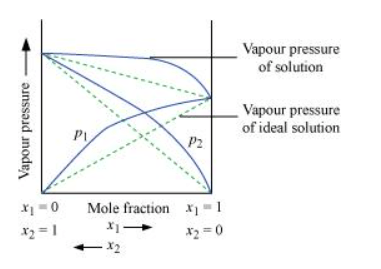Vapour pressure of a two-component solution showing positive deviation from Raoult’s law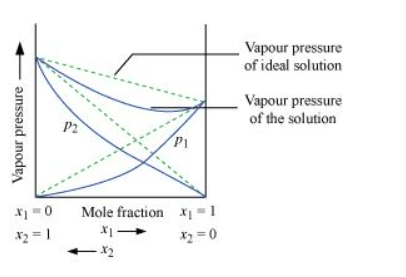Vapour pressure of a two-component solution showing negative deviation from Raoult’s law

In the case of an ideal solution, the enthalpy of the mixing of the pure components for forming the solution is zero.

$\Delta_{\mathrm{sol}} H=0$

In the case of solutions showing positive deviations, absorption of heat takes place.

$\therefore \Delta_{\mathrm{Sol}} H=$ Positive

In the case of solutions showing negative deviations, evolution of heat takes place.

$\therefore \Delta_{\text {sol }} H=$ Negative

Question 15: An aqueous solution of $2 \%$ non-volatile solute exerts a pressure of $1.004$ bar at the normal boiling point of the solvent. What is the molar mass of the solute?

Solution. Here,

Vapour pressure of the solution at normal boiling point $\left(p_{1}\right)=1.004$ bar

Vapour pressure of pure water at normal boiling point $\left(p_{1}^{0}\right)=1.013$ bar

Mass of solute, $\left(w_{2}\right)=2 \mathrm{~g}$

Mass of solvent (water), $\left(w_{1}\right)=98 \mathrm{~g}$

Molar mass of solvent (water), $\left(M_{1}\right)=18 \mathrm{~g} \mathrm{~mol}^{-1}$

According to Raoult’s law,

$\frac{p_{1}^{0}-p_{1}}{p_{1}^{0}}=\frac{w_{2} \times M_{1}}{M_{2} \times w_{1}}$

$\Rightarrow \frac{1.013-1.004}{1.013}=\frac{2 \times 18}{M_{2} \times 98}$

$\Rightarrow \frac{0.009}{1.013}=\frac{2 \times 18}{M_{2} \times 98}$

$\Rightarrow \frac{0.009}{1.013}=\frac{2 \times 18}{M_{2} \times 98}$

$\Rightarrow M_{2}=\frac{1.013 \times 2 \times 18}{0.009 \times 98}$

$=41.35 \mathrm{~g} \mathrm{~mol}^{-1}$

Hence, the molar mass of the solute is $41.35 \mathrm{~g} \mathrm{~mol}^{-1}$.

Question 16: Heptane and octane form an ideal solution. At $373 \mathrm{~K}$, the vapour pressures of the two liquid components are $105.2 \mathrm{kPa}$ and $46.8 \mathrm{kPa}$ respectively. What will be the vapour pressure of a mixture of $26.0 \mathrm{~g}$ of heptane and $35 \mathrm{~g}$ of octane?

Solution. Vapour pressure of heptane $\left(p_{1}^{0}\right)=105.2 \mathrm{kPa}$

Vapour pressure of octane $\left(p_{2}^{0}\right)=46.8 \mathrm{kPa}$

We know that,

Molar mass of heptane $\left(\mathrm{C}_{7} \mathrm{H}_{16}\right)=7 \times 12+16 \times 1$

$=100 \mathrm{~g} \mathrm{~mol}^{-1}$

$\therefore$ Number of moles of heptane $=\frac{26}{100} \mathrm{~mol}$

$=0.26 \mathrm{~mol}$

Molar mass of octane $\left(\mathrm{C}_{8} \mathrm{H}_{18}\right)=8 \times 12+18 \times 1$

$=114 \mathrm{~g} \mathrm{~mol}^{-1}$

$\therefore$ Number of moles of octane $=\frac{35}{114}$ mol

$=0.31 \mathrm{~mol}$

Mole fraction of heptane, $x_{1}=\frac{0.26}{0.26+0.31}$

$=0.456$

And, mole fraction of octane, $x_{2}=1-0.456$

$=0.544$

Now, partial pressure of heptane, $p_{1}=x_{1} p_{1}^{0}$

$=0.456 \times 105.2$

$=47.97 \mathrm{kPa}$

Partial pressure of octane, $p_{2}=x_{2} p_{2}^{0}$

$=0.544 \times 46.8$

$=25.46 \mathrm{kPa}$

Hence, vapour pressure of solution, $p_{\text {total }}=p_{1}+p_{2}$

$=47.97+25.46$

$=73.43 \mathrm{kPa}$

Question 17: The vapour pressure of water is $12.3 \mathrm{kPa}$ at $300 \mathrm{~K}$. Calculate vapour pressure of 1 molal solution of a non-volatile solute in it.

Solution. 1 molal solution means 1 mol of the solute is present in 1000 g of the solvent (water).

Molar mass of water $=18 \mathrm{~g} \mathrm{~mol}^{-1}$

$\therefore$ Number of moles present in $1000 \mathrm{~g}$ of water $=\frac{1000}{18}$

$=55.56 \mathrm{~mol}$

Therefore, mole fraction of the solute in the solution is

$x_{2}=\frac{1}{1+55.56}=0.0177$

It is given that,

Vapour pressure of water, $p_{1}^{0}=12.3 \mathrm{kPa}$

Applying the relation, $\frac{p_{1}^{0}-p_{1}}{p_{1}^{0}}=x_{2}$

$\Rightarrow \frac{12.3-p_{1}}{12.3}=0.0177$

$\Rightarrow 12.3-p_{1}=0.2177$

$\Rightarrow p_{1}=12.0823$

$=12.08 \mathrm{kPa}$ (approximately)

Hence, the vapour pressure of the solution is $12.08 \mathrm{kPa}$.

Question 18: Calculate the mass of a non-volatile solute (molar mass $\left.40 \mathrm{~g} \mathrm{~mol}^{-1}\right)$ which should be dissolved in 114 g octane to reduce its vapour pressure to $80 \%$

Solution. Let the vapour pressure of pure octane be $p_{1}^{0}$.

Then, the vapour pressure of the octane after dissolving the non-volatile solute is $\frac{80}{100} p_{1}^{0}=0.8 p_{1}^{0}$.

Molar mass of solute, $M_{2}=40 \mathrm{~g} \mathrm{~mol}^{-1}$

Mass of octane, $w_{1}=114 \mathrm{~g}$

Molar mass of octane, $\left(\mathrm{C}_{8} \mathrm{H}_{18}\right), M_{1}=8 \times 12+18 \times 1$

$=114 \mathrm{~g} \mathrm{~mol}^{-1}$

Applying the relation,

$\frac{p_{1}^{0}-p_{1}}{p_{1}^{0}}=\frac{w_{2} \times M_{1}}{M_{2} \times w_{1}}$

$\Rightarrow \frac{p_{1}^{0}-0.8 p_{1}^{0}}{p_{1}^{0}}=\frac{w_{2} \times 114}{40 \times 114}$

$\Rightarrow \frac{0.2 p_{1}^{0}}{p_{1}^{0}}=\frac{w_{2}}{40}$

$\Rightarrow 0.2=\frac{w_{2}}{40}$

$\Rightarrow w_{2}=8 \mathrm{~g}$

Hence, the required mass of the solute is 8 g.

Question 19: A solution containing $30 \mathrm{~g}$ of non-volatile solute exactly in $90 \mathrm{~g}$ of water has a

vapour pressure of $2.8 \mathrm{kPa}$ at $298 \mathrm{~K}$. Further, $18 \mathrm{~g}$ of water is then added to

the solution and the new vapour pressure becomes $2.9 \mathrm{kPa}$ at $298 \mathrm{~K}$. Calculate:

i. molar mass of the solute

ii. vapour pressure of water at $298 \mathrm{~K}$.

Solution. (i) Let, the molar mass of the solute be $\mathrm{M} \mathrm{g} \mathrm{mol}^{-1}$

Now, the no. of moles of solvent (water), $n_{1}=\frac{90 \mathrm{~g}}{18 \mathrm{~g} \mathrm{~mol}^{-1}}=5 \mathrm{~mol}$

And, the no. of moles of solute, $n_{2}=\frac{30 \mathrm{~g}}{\mathrm{M} \mathrm{mol}^{-1}}=\frac{30}{\mathrm{M}} \mathrm{mo}$

$p_{1}=2.8 \mathrm{kPa}$

Applying the relation:

$\frac{p_{1}^{0}-p_{1}}{p_{1}^{0}}=\frac{n_{2}}{n_{1}+n_{2}}$

$\Rightarrow \frac{p_{1}^{0}-2.8}{p_{1}^{0}}=\frac{\frac{30}{\mathrm{M}}}{5+\frac{30}{\mathrm{M}}}$

$\Rightarrow 1-\frac{2.8}{p_{1}^{0}}=\frac{\frac{30}{\mathrm{M}}}{\frac{5 \mathrm{M}+30}{\mathrm{M}}}$

$\Rightarrow 1-\frac{2.8}{p_{1}^{0}}=\frac{30}{5 \mathrm{M}+30}$

$\Rightarrow \frac{2.8}{p_{1}^{0}}=1-\frac{30}{5 \mathrm{M}+30}$

$\Rightarrow \frac{2.8}{p_{1}^{0}}=\frac{5 \mathrm{M}+30-30}{5 \mathrm{M}+30}$

$\Rightarrow \frac{2.8}{p_{1}^{0}}=\frac{5 \mathrm{M}}{5 \mathrm{M}+30}$

$\Rightarrow \frac{p_{1}^{0}}{2.8}=\frac{5 \mathrm{M}+30}{5 \mathrm{M}}$$(i) After the addition of 18 g of water: n_{1}=\frac{90+18 \mathrm{~g}}{18}=6 \mathrm{~mol} p_{1}=2.9 \mathrm{kPa} Again, applying the relation: \frac{p_{1}^{0}-p_{1}}{p_{1}^{0}}=\frac{n_{2}}{n_{1}+n_{2}} \Rightarrow \frac{p_{1}^{0}-2.9}{p_{1}^{0}}=\frac{\frac{30}{\mathrm{M}}}{6+\frac{30}{\mathrm{M}}} \Rightarrow 1-\frac{2.9}{p_{1}^{9}}=\frac{\frac{30}{\mathrm{M}}}{\frac{6 \mathrm{M}+30}{\mathrm{M}}} \Rightarrow 1-\frac{2.9}{p_{1}^{0}}=\frac{30}{6 \mathrm{M}+30} \Rightarrow \frac{2.9}{p_{1}^{0}}=1-\frac{30}{6 \mathrm{M}+30} \Rightarrow \frac{2.9}{p_{1}^{0}}=\frac{6 \mathrm{M}+30-30}{6 \mathrm{M}+30} \Rightarrow \frac{2.9}{p_{1}^{0}}=\frac{6 \mathrm{M}}{6 \mathrm{M}+30} \Rightarrow \frac{p_{1}^{0}}{2.9}=\frac{6 \mathrm{M}+30}{6 \mathrm{M}}$$(i i)$

Dividing equation (i) by (ii), we have:

$\frac{2.9}{2.8}=\frac{\frac{5 \mathrm{M}+30}{5 \mathrm{M}}}{\frac{6 \mathrm{M}+30}{6 \mathrm{M}}}$

$\Rightarrow \frac{2.9}{2.8} \times \frac{6 \mathrm{M}+30}{6}=\frac{5 \mathrm{M}+30}{5}$

$\Rightarrow 2.9 \times 5 \times(6 \mathrm{M}+30)=2.8 \times 6 \times(5 \mathrm{M}+30)$

$\Rightarrow 87 \mathrm{M}+435=84 \mathrm{M}+504$

$\Rightarrow 3 \mathrm{M}=69$

$\Rightarrow \mathrm{M}=23 \mathrm{u}$

Therefore, the molar mass of the solute is $23 \mathrm{~g} \mathrm{~mol}^{-1}$.

(ii) Putting the value of ' $\mathrm{M}$ ' in equation (i), we have:

$\frac{p_{1}^{0}}{2.8}=\frac{5 \times 23+30}{5 \times 23}$

$\Rightarrow \frac{p_{1}^{0}}{2.8}=\frac{145}{115}$

$\Rightarrow p_{1}^{0}=3.53$

Hence, the vapour pressure of water at $298 \mathrm{~K}$ is $3.53 \mathrm{kPa}$.

Question 20: A $5 \%$ solution (by mass) of cane sugar in water has freezing point of $271 \mathrm{~K} .$ Calculate the freezing point of $5 \%$ glucose in water if freezing point of pure water is $273.15 \mathrm{~K}$.

Solution. Here, $\Delta T_{f}=(273.15-271) \mathrm{K}$

$=2.15 \mathrm{~K}$

Molar mass of sugar $\left(\mathrm{C}_{12} \mathrm{H}_{22} \mathrm{O}_{11}\right)=12 \times 12+22 \times 1+11 \times 16$

$=342 \mathrm{~g} \mathrm{~mol}^{-1}$

$5 \%$ solution (by mass) of cane sugar in water means $5 \mathrm{~g}$ of cane sugar is present in $(100-5) \mathrm{g}=95 \mathrm{~g}$ of water.

Now, number of moles of cane sugar $=\frac{5}{342}$ mol

$=0.0146 \mathrm{~mol}$

Therefore, molality of the solution, $m=\frac{0.0146 \mathrm{~mol}}{0.095 \mathrm{~kg}}$

$=0.1537 \mathrm{~mol} \mathrm{~kg}^{-1}$

Applying the relation,

$\Delta T_{f}=K_{f} \times m$

$\Delta T_{f}=K_{f} \times m$

$\Rightarrow K_{f}=\frac{\Delta T_{f}}{m}$

$=\frac{2.15 \mathrm{~K}}{0.1537 \mathrm{~mol} \mathrm{~kg}^{-1}}$

$=13.99 \mathrm{~K} \mathrm{~kg} \mathrm{~mol}^{-1}$

$5 \%$ glucose in water means $5 \mathrm{~g}$ of glucose is present in $(100-5) \mathrm{g}=95 \mathrm{~g}$ of water.

$\therefore$ Number of moles of glucose $=\frac{5}{180}$ mol

$=0.0278 \mathrm{~mol}$

Therefore, molality of the solution, $m=\frac{0.0278 \mathrm{~mol}}{0.095 \mathrm{~kg}}$

$=0.2926 \mathrm{~mol} \mathrm{~kg}^{-1}$

Applying the relation,

$\Delta T_{f}=K_{f} \times m$

$=13.99 \mathrm{~K} \mathrm{~kg} \mathrm{~mol}^{-1} \times 0.2926 \mathrm{~mol} \mathrm{~kg}^{-1}$

$=4.09 \mathrm{~K}$ (approximately)

Hence, the freezing point of $5 \%$ glucose solution is $(273.15-4.09) \mathrm{K}=269.06 \mathrm{~K}$.

Question $21$ Two elements $A$ and $B$ form compounds having formula $A B_{2}$ and $A B_{4} .$ When dissolved in 20 g of benzene $\left(C_{6} H_{6}\right), 1 \mathrm{~g}$ of $A B_{2}$ lowers the freezing point by $2.3$ Kwhereas $1.0 \mathrm{~g}$ of $\mathrm{AB}_{4}$ lowers it by $1.3 \mathrm{~K}$. The molar depression constant for benzene is $5.1 \mathrm{Kkg} \mathrm{mol}^{-1} .$ Calculate atomic masses of $\mathrm{A}$ and $\mathrm{B}$.

Solution. We know that,

$M_{2}=\frac{1000 \times w_{2} \times k_{f}}{\Delta T_{f} \times w_{1}}$

Then, $M_{\mathrm{AB}_{2}}=\frac{1000 \times 1 \times 5.1}{2.3 \times 20}$

$=110.87 \mathrm{~g} \mathrm{~mol}^{-1}$

$M_{A B_{4}}=\frac{1000 \times 1 \times 5.1}{1.3 \times 20}$

$M_{\mathrm{AB}_{4}}=\frac{1000 \times 1 \times 5.1}{1.3 \times 20}$

$=196.15 \mathrm{~g} \mathrm{~mol}^{-1}$

Now, we have the molar masses of $A B_{2}$ and $A B_{4}$ as $110.87 \mathrm{~g} \mathrm{~mol}^{-1}$ and $196.15 \mathrm{~g} \mathrm{~mol}^{-1}$ respectively.

Let the atomic masses of $A$ and $B$ be $x$ and $y$ respectively.

Now, we can write:

$x+2 y=110.87$(i)

$x+4 y=196.15 \quad$ (ii)

Subtracting equation (i) from (ii), we have

$2 y=85.28$

$\Rightarrow y=42.64$

Putting the value of ' $y$ ' in equation (1), we have

$x+2 \times 42.64=110.87$

$\Rightarrow x=25.59$

Hence, the atomic masses of $A$ and $B$ are $25.59 u$ and $42.64 u$ respectively.

Question 22: At $300 \mathrm{~K}, 36 \mathrm{~g}$ of glucose present in a litre of its solution has an osmotic pressure of $4.98$ bar. If the osmotic pressure of the solution is $1.52$ bars at the same temperature, what would be its concentration?

Solution. Here,

$T=300 \mathrm{~K}$

$T=300 \mathrm{~K}$

$\pi=1.52$ bar

$\mathrm{R}=0.083 \mathrm{bar} \mathrm{L} \mathrm{K}^{-1} \mathrm{~mol}^{-1}$

Applying the relation,

$\Pi=C R T$

$\Rightarrow C=\frac{\pi}{\mathrm{R} T}$

$=\frac{1.52 \text { bar }}{0.083 \text { bar } L K^{-1} m o l^{-1} \times 300 K}$

$=0.061 \mathrm{~mol}$

Since the volume of the solution is $1 \mathrm{~L}$, the concentration of the solution would be $0.061 \mathrm{M}$.

Question 23: Suggest the most important type of intermolecular attractive interaction in the following pairs.

(i) n-hexane and n-octane

(ii) $\mathrm{I}_{2}$ and $\mathrm{CCl}_{4}$

(iii) $\mathrm{NaClO}_{4}$ and water

(iv) methanol and acetone

(v) acetonitrile $\left(\mathrm{CH}_{3} \mathrm{CN}\right)$ and acetone $\left(\mathrm{C}_{3} \mathrm{H}_{6} \mathrm{O}\right)$.

Solution. (i) Van der Wall's forces of attraction.

(ii) Van der Wall’s forces of attraction.

(iii) Ion-diople interaction.

(iv) Dipole-dipole interaction.

(v) Dipole-dipole interaction.

Question 24: Based on solute-solvent interactions, arrange the following in order of increasing solubility in n-octane and explain. Cyclohexane, $\mathrm{KCl}, \mathrm{CH}_{3} \mathrm{OH}$, $\mathrm{CH}_{3} \mathrm{CN}$

Solution. n-octane is a non-polar solvent. Therefore, the solubility of a non-polar solute is more than that of a polar solute in the $n$-octane.

The order of increasing polarity is:

Cyclohexane $<\mathrm{CH}_{3} \mathrm{CN}<\mathrm{CH}_{3} \mathrm{OH}<\mathrm{KCl}$

Therefore, the order of increasing solubility is:

$\mathrm{KCl}<\mathrm{CH}_{3} \mathrm{OH}<\mathrm{CH}_{3} \mathrm{CN}<$ Cyclohexane

Question 25: Amongst the following compounds, identify which are insoluble, partially soluble and highly soluble in water?

(i) phenol

(ii) toluene

(iii) formic acid

(iv) ethylene glycol

(v) chloroform

(vi) pentanol.

Solution. (i) Phenol $\left(\mathrm{C}_{6} \mathrm{H}_{5} \mathrm{OH}\right)$ has the polar group $-\mathrm{OH}$ and non-polar group $-\mathrm{C}_{6} \mathrm{H}_{5}$. Thus, phenol is partially soluble in water.

(ii) Toluene $\left(\mathrm{C}_{6} \mathrm{H}_{5}-\mathrm{CH}_{3}\right)$ has no polar groups. Thus, toluene is insoluble in water.

(iii) Formic acid (HCOOH) has the polar group -OH and can form H-bond with water. Thus, formic acid is highly soluble in water.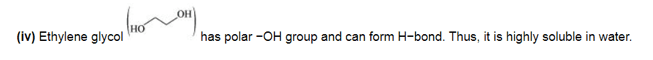(v) Chloroform is insoluble in water.

(vi) Pentanol $\left(\mathrm{C}_{5} \mathrm{H}_{11} \mathrm{OH}\right)$ has polar -OH group, but it also contains a very bulky non-polar $-\mathrm{C}_{5} \mathrm{H}_{11}$ group. Thus, pentanol is partially soluble in water.

Question 26: If the density of some lake water is $1.25 \mathrm{~g} \mathrm{~mL}^{-1}$ and contains $92 \mathrm{~g}$ of $\mathrm{Na}^{+}$ions per $\mathrm{kg}$ of water, calculate the molality of $\mathrm{Na}^{+}$ions in the lake.

Solution. Number of moles present in $92 \mathrm{~g}$ of $\mathrm{Na}^{+}$ions $=\frac{92 \mathrm{~g}}{23 \mathrm{~g} \mathrm{~mol}^{-1}}$

$=4 \mathrm{~mol}$

Therefore, molality of $\mathrm{Na}^{+}$ions in the lake $=\frac{4 \mathrm{~mol}}{1 \mathrm{~kg}}$

$=4 \mathrm{~m}$

Question 27: If the solubility product of CuS is $6 \times 10^{-16}$, calculate the maximum molarity of CuS in aqueous solution.

Solution. Solubility product of $\mathrm{CuS}, K_{\mathrm{sp}}=6 \times 10^{-16}$

Let $s$ be the solubility of CuS in mol $L^{-1}$.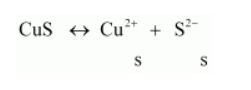Now, $K_{i p}=\left[\mathrm{Cu}^{2+}\right]\left[\mathrm{S}^{2-}\right]$

$=s \times s$

$=s^{2}$

Then, we have, $K_{\mathrm{sp}}=s^{2}=6 \times 10^{-16}$

$\Rightarrow s=\sqrt{6 \times 10^{-16}}$

$=2.45 \times 10^{-8} \mathrm{~mol} \mathrm{~L}^{-1}$

Hence, the maximum molarity of CuS in an aqueous solution is $2.45 \times 10^{-8} \mathrm{~mol} \mathrm{~L}^{-1}$.

Question 28: Calculate the mass percentage of aspirin $\left(\mathrm{C}_{9} \mathrm{H}_{8} \mathrm{O}_{4}\right)$ in acetonitrile $\left(\mathrm{CH}_{3} \mathrm{CN}\right)$ when $6.5 \mathrm{~g}$ of $\mathrm{C}_{9} \mathrm{H}_{8} \mathrm{O}_{4}$ is dissolved in $450 \mathrm{~g}$ of $\mathrm{CH}_{3} \mathrm{CN}$.

Solution. $6.5 \mathrm{~g}$ of $\mathrm{C}_{9} \mathrm{H}_{8} \mathrm{O}_{4}$ is dissolved in $450 \mathrm{~g}$ of $\mathrm{CH}_{3} \mathrm{CN}$.

Then, total mass of the solution $=(6.5+450) \mathrm{g}$

$=456.5 \mathrm{~g}$

Therefore, mass percentage of $\mathrm{C}_{9} \mathrm{H}_{8} \mathrm{O}_{4}=\frac{6.5}{456.5} \times 100 \%$

$=1.424 \%$

Question 29: Nalorphene $\left(\mathrm{C}_{19} \mathrm{H}_{21} \mathrm{NO}_{3}\right)$, similar to morphine, is used to combat withdrawal

symptoms in narcotic users. Dose of nalorphene generally given is $1.5 \mathrm{mg}$.

Calculate the mass of $1.5 \times 10^{-3} \mathrm{~m}$ aqueous solution required for the above dose.

Solution. The molar mass of nalorphene $\left(\mathrm{C}_{19} \mathrm{H}_{21} \mathrm{NO}_{3}\right)$ is given as:

$19 \times 12+21 \times 1+1 \times 14+3 \times 16=311 \mathrm{~g} \mathrm{~mol}^{-1}$

In $1.5 \times 10^{-3} \mathrm{~m}$ aqueous solution of nalorphene,

$1 \mathrm{~kg}(1000 \mathrm{~g})$ of water contains $1.5 \times 10^{-3} \mathrm{~mol}=1.5 \times 10^{-3} \times 311 \mathrm{~g}$

$=0.4665 \mathrm{~g}$

Therefore, total mass of the solution $=(1000+0.4665) \mathrm{g}$

$=1000.4665 \mathrm{~g}$

This implies that the mass of the solution containing $0.4665 \mathrm{~g}$ of nalorphene is $1000.4665 \mathrm{~g}$.

Therefore, mass of the solution containing $1.5 \mathrm{mg}$ of nalorphene is:

$\frac{1000.4665 \times 1.5 \times 10^{-3}}{0.4665} \mathrm{~g}$

$=3.22 \mathrm{~g}$

Hence, the mass of aqueous solution required is $3.22 \mathrm{~g}$.

Note: There is a slight variation in this answer and the one given in the NCERT textbook.

Question 30: Calculate the amount of benzoic acid $\left(\mathrm{C}_{6} \mathrm{H}_{5} \mathrm{COOH}\right)$ required for preparing $250 \mathrm{~mL}$ of $0.15 \mathrm{M}$ solution in methanol.

Solution. $0.15 \mathrm{M}$ solution of benzoic acid in methanol means,

$1000 \mathrm{~mL}$ of solution contains $0.15 \mathrm{~mol}$ of benzoic acid

Therefore, $250 \mathrm{~mL}$ of solution contains $=\frac{0.15 \times 250}{1000}$ mol of benzoic acid

$=0.0375$ mol of benzoic acid

Molar mass of benzoic acid $\left(\mathrm{C}_{6} \mathrm{H}_{5} \mathrm{COOH}\right)=7 \times 12+6 \times 1+2 \times 16$

$=122 \mathrm{~g} \mathrm{~mol}^{-1}$

Molar mass of benzoic acid $\left(\mathrm{C}_{6} \mathrm{H}_{5} \mathrm{COOH}\right)=7 \times 12+6 \times 1+2 \times 16$

$=122 \mathrm{~g} \mathrm{~mol}^{-1}$

Hence, required benzoic acid $=0.0375 \mathrm{~mol} \times 122 \mathrm{~g} \mathrm{~mol}^{-1}$

$=4.575 \mathrm{~g}$

Question 31: The depression in freezing point of water observed for the same amount of acetic acid, trichloroacetic acid and trifluoroacetic acid increases in the order given above. Explain briefly.

Solution.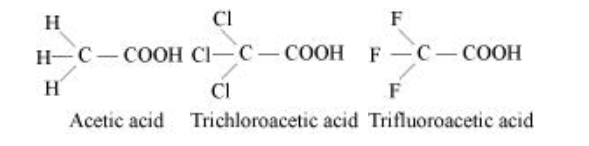Among H, Cl, and F, H is least electronegative while F is most electronegative. Then, F can withdraw electrons towards itself more than Cl and H. Thus, trifluoroacetic acid can easily lose H+ ions i.e., trifluoroacetic acid ionizes to the largest extent. Now, the more ions produced, the greater is the depression of the freezing point. Hence, the depression in the freezing point increases in the order:

Acetic acid < trichloroacetic acid $<$ trifluoroacetic acid

Question 32: Calculate the depression in the freezing point of water when $10 \mathrm{~g}$ of $\mathrm{CH}_{3} \mathrm{CH}_{2} \mathrm{CHClCOOH}$ is added to $250 \mathrm{~g}$ of water. $K_{a}=1.4 \times 10^{-3}, K_{f}=1.86$

$\mathrm{K} \mathrm{kg} \mathrm{mol}^{-1}$.

Solution. Molar mass of $\mathrm{CH}_{3} \mathrm{CH}_{2} \mathrm{CHClCOOH}=15+14+13+35.5+12+16+16+1$

$=122.5 \mathrm{~g} \mathrm{~mol}^{-1}$

$\therefore$ No. of moles present in $10 \mathrm{~g}$ of $\mathrm{CH}_{3} \mathrm{CH}_{2} \mathrm{CHClCOOH}=\frac{10 \mathrm{~g}}{122.5 \mathrm{~g} \mathrm{~mol}^{-1}}$

$=0.0816 \mathrm{~mol}$

It is given that $10 \mathrm{~g}$ of $\mathrm{CH}_{3} \mathrm{CH}_{2} \mathrm{CHClCOOH}$ is added to $250 \mathrm{~g}$ of water.

$\therefore$ Molality of the solution, $=\frac{0.0186}{250} \times 1000$

$=0.3264 \mathrm{~mol} \mathrm{~kg}^{-1}$

Let $\alpha$ be the degree of dissociation of $\mathrm{CH}_{3} \mathrm{CH}_{2} \mathrm{CHClCOOH}$.

$\mathrm{CH}_{3} \mathrm{CH}_{2} \mathrm{CHClCOOH}$ undergoes dissociation according to the following equation: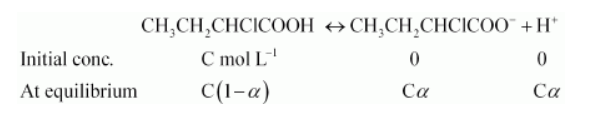$\therefore K_{a}=\frac{C \alpha \cdot C \alpha}{C(1-\alpha)}$

$=\frac{C \alpha^{2}}{1-\alpha}$

Since $\alpha$ is very small with respect to $1,1-a \approx 1$

Now, $K_{a}=\frac{C \alpha^{2}}{1}$

$\Rightarrow K_{a}=C \alpha^{2}$

$\Rightarrow \alpha=\sqrt{\frac{K_{a}}{C}}$

$=\sqrt{\frac{1.4 \times 10^{-3}}{0.3264}} \quad\left(\because K_{a}=1.4 \times 10^{-3}\right)$

$=0.0655$

Again,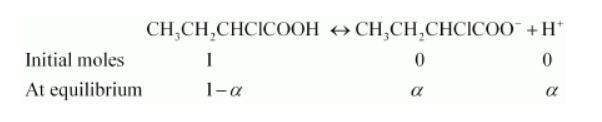Total moles of equilibrium $=1-\alpha+a+a$

$=1+\alpha$

$\therefore i=\frac{1+\alpha}{1}$

$=1+\alpha$

$=1+0.0655$

$=1.0655$

Hence, the depression in the freezing point of water is given as:

$\Delta T_{f}=i \cdot K_{f} m$

$=1.0655 \times 1.86 \mathrm{~K} \mathrm{~kg} \mathrm{~mol}^{-1} \times 0.3264 \mathrm{~mol} \mathrm{~kg}^{-1}$

$=0.65 \mathrm{~K}$ Question 33: $19.5 \mathrm{~g}$ of $\mathrm{CH}_{2}$ FCOOH is dissolved in $500 \mathrm{~g}$ of water. The depression in the freezing point of water observed is $1.0^{\circ} \mathrm{C} .$ Calculate the van't Hoff factor and dissociation constant of fluoroacetic acid.

Solution. It is given that:

$w_{1}=500 \mathrm{~g}$

$w_{2}=19.5 \mathrm{~g}$

$K_{f}=1.86 \mathrm{~K} \mathrm{~kg} \mathrm{~mol}^{-1}$

$\Delta T_{f}=1 \mathrm{~K}$

We know that:

$M_{2}=\frac{K_{f} \times w_{2} \times 1000}{\Delta T_{f} \times w_{1}}$

$=\frac{1.86 \mathrm{Kkg} \mathrm{mol}^{-1} \times 19.5 \mathrm{~g} \times 1000 \mathrm{~g} \mathrm{~kg}^{-1}}{500 \mathrm{~g} \times 1 \mathrm{~K}}$

$=72.54 \mathrm{~g} \mathrm{~mol}^{-1}$

Therefore, observed molar mass of $\mathrm{CH}_{2} \mathrm{FCOOH},\left(M_{2}\right)_{\text {obs }}=72.54 \mathrm{~g} \mathrm{~mol}$

The calculated molar mass of $\mathrm{CH}_{2} \mathrm{FCOOH}$ is:

$\left(M_{2}\right)_{\mathrm{cal}}=14+19+12+16+16+1$

$=78 \mathrm{~g} \mathrm{~mol}^{-1}$

Therefore, van't Hoff factor, $i=\frac{\left(M_{2}\right)_{\mathrm{cal}}}{\left(M_{2}\right)_{\text {obs }}}$

$=\frac{78 \mathrm{~g} \mathrm{~mol}^{-1}}{72.54 \mathrm{~g} \mathrm{~mol}^{-1}}$

$=1.0753$

Let $a$ be the degree of dissociation of $\mathrm{CH}_{2} \mathrm{FCOOH}$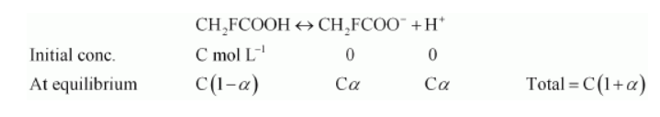$\therefore i=\frac{C(1+\alpha)}{C}$

$\Rightarrow i=1+\alpha$

$\Rightarrow \alpha=i-1$

$=1.0753-1$

$=0.0753$

Now, the value of $K_{a}$ is given as:

$K_{a}=\frac{\left[\mathrm{CH}_{2} \mathrm{FCOO}^{-}\right]\left[\mathrm{H}^{+}\right]}{\left[\mathrm{CH}_{2} \mathrm{FCOOH}\right]}$

$=\frac{C \alpha \cdot C \alpha}{C(1-\alpha)}$

$=\frac{C \alpha^{2}}{1-\alpha}$

Taking the volume of the solution as $500 \mathrm{~mL}$, we have the concentration:

$C=\frac{\frac{19.5}{78}}{500} \times 1000 \mathrm{M}$

$=0.5 \mathrm{M}$

Therefore, $K_{a}=\frac{C \alpha^{2}}{1-\alpha}$

$=\frac{0.5 \times(0.0753)^{2}}{1-0.0753}$

$=\frac{0.5 \times 0.00567}{0.9247}$

$=0.00307$ (approximately)

$=3.07 \times 10^{-3}$

Question 34: Vapour pressure of water at 293 Kis $17.535 \mathrm{~mm} \mathrm{Hg} .$ Calculate the vapour pressure of water at 293 Kwhen $25 \mathrm{~g}$ of glucose is dissolved in 450 $\mathrm{g}$ of water.

Solution. Vapour pressure of water, $p_{1}^{0}=17.535 \mathrm{~mm}$ of $\mathrm{Hg}$

Mass of glucose, $w_{2}=25 \mathrm{~g}$

Mass of water, $w_{1}=450 \mathrm{~g}$

We know that,

Molar mass of glucose $\left(\mathrm{C}_{6} \mathrm{H}_{12} \mathrm{O}_{6}\right), M_{2}=6 \times 12+12 \times 1+6 \times 16$

$=180 \mathrm{~g} \mathrm{~mol}^{-1}$

Molar mass of water, $M_{1}=18 \mathrm{~g} \mathrm{~mol}^{-1}$

Then, number of moles of glucose, $n_{2}=\frac{25}{180 \mathrm{~g} \mathrm{~mol}^{-1}}$

$=0.139 \mathrm{~mol}$

And, number of moles of water, $n_{1}=\frac{450 \mathrm{~g}}{18 \mathrm{~g} \mathrm{~mol}^{-1}}$

$=25 \mathrm{~mol}$

We know that,

$\frac{p_{1}^{0}-p_{1}}{p_{1}^{0}}=\frac{n_{1}}{n_{2}+n_{1}}$

$\Rightarrow \frac{17.535-p_{1}}{17.535}=\frac{0.139}{0.139+25}$

$\Rightarrow 17.535-p_{1}=\frac{0.139 \times 17.535}{25.139}$

$\Rightarrow 17.535-p_{1}=0.097$

$\Rightarrow p_{1}=17.44 \mathrm{~mm}$ of $\mathrm{Hg}$

Hence, the vapour pressure of water is $17.44 \mathrm{~mm}$ of $\mathrm{Hg}$.

Question $35$ : Henry's law constant for the molality of methane in benzene at 298 Kis $4.27 \times 10^{5} \mathrm{~mm} \mathrm{Hg} .$ Calculate the solubility of methane in benzene at 298 Kunder $760 \mathrm{~mm} \mathrm{Hg}$.

Solution. Here,

$p=760 \mathrm{~mm} \mathrm{Hg}$

$\mathrm{k}_{\mathrm{H}}=4.27 \times 10^{5} \mathrm{~mm} \mathrm{Hg}$

According to Henry's law,

$p=\mathrm{k}_{\mathrm{H}} x$

$\Rightarrow x=\frac{p}{k_{\mathrm{H}}}$

$=\frac{760 \mathrm{~mm} \mathrm{Hg}}{4.27 \times 10^{5} \mathrm{~mm} \mathrm{Hg}}$

$=177.99 \times 10^{-5}$

$=178 \times 10^{-5}$ (approximately)

Hence, the mole fraction of methane in benzene is $178 \times 10^{-5}$.

Question 36: $100 \mathrm{~g}$ of liquid $\mathrm{A}$ (molar mass $\left.140 \mathrm{~g} \mathrm{~mol}^{-1}\right)$ was dissolved in $1000 \mathrm{~g}$ of liquid $\mathrm{B}$ (molar mass $\left.180 \mathrm{~g} \mathrm{~mol}^{-1}\right) .$ The vapour pressure of pure liquid $\mathrm{B}$ was found to be 500 torr. Calculate the vapour pressure of pure liquid A and its vapour pressure in the solution if the total vapour pressure of the solution is 475 Torr.

Solution. Number of moles of liquid $\mathrm{A}, n_{\mathrm{A}}=\frac{100}{140} \mathrm{~mol}$

$=0.714 \mathrm{~mol}$

Number of moles of liquid B, $n_{\mathrm{B}}=\frac{1000}{180}$ mol

$=5.556 \mathrm{~mol}$

Then, mole fraction of $\mathrm{A}, x_{\mathrm{A}}=\frac{n_{\mathrm{A}}}{n_{\mathrm{A}}+n_{\mathrm{B}}}$

$=\frac{0.714}{0.714+5.556}$

$=0.114$

And, mole fraction of $B, x_{B}=1-0.114$

$=0.886$

Vapour pressure of pure liquid $B, p_{\mathrm{B}}^{0}=500$ torr

Therefore, vapour pressure of liquid $\mathrm{B}$ in the solution,

$p_{\mathrm{B}}=p_{\mathrm{B}}^{0} x_{\mathrm{B}}$

$=500 \times 0.886$

$=443$ torr

Total vapour pressure of the solution, $p_{\text {total }}=475$ torr

$\therefore$ Vapour pressure of liquid $\mathrm{A}$ in the solution,

$p_{\mathrm{A}}=p_{\text {total }}-p_{\mathrm{B}}$

$=475-443$

$=32$ torr

Now,

$p_{\mathrm{A}}=p_{\mathrm{A}}^{0} x_{\mathrm{A}}$

$\Rightarrow p_{\mathrm{A}}^{0}=\frac{p_{\mathrm{A}}}{x_{\mathrm{A}}}$

$=\frac{32}{0.114}$

$=280.7$ torr

Hence, the vapour pressure of pure liquid $\mathrm{A}$ is $280.7$ torr.

Question $37:$ Vapour pressure of pure acetone and chloroform at $328 \mathrm{~K}$ are $741.8 \mathrm{~mm} \mathrm{Hg}$ and $632.8 \mathrm{~mm} \mathrm{Hg}$ respectively. Assuming that they form ideal solution over the entire range of composition, plot $p_{\text {total }} P_{\text {chloroform' }}$ and $p_{\text {acetone }}$ as a function of $x_{\text {acetone }}$. The experimental data observed for different compositions of mixture is.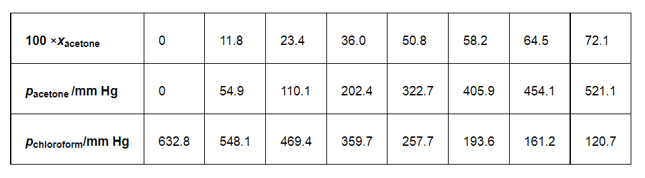Solution. From the question, we have the following data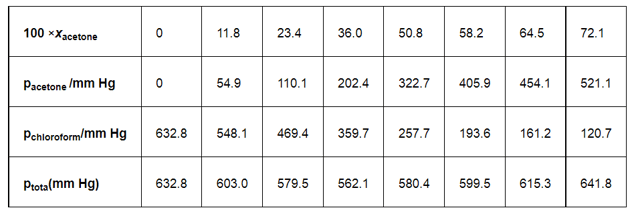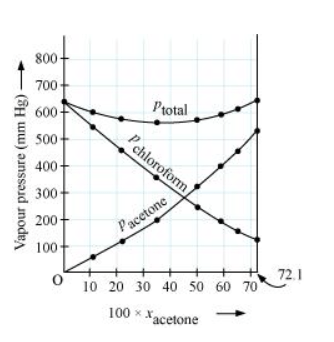It can be observed from the graph that the plot for the $p_{\text {total }}$ of the solution curves downwards. Therefore, the solution shows negative deviation from the ideal behavior.

Question 38: Benzene and toluene form ideal solution over the entire range of composition. The vapour pressure of pure benzene and toluene at $300 \mathrm{~K}$ are $50.71 \mathrm{~mm} \mathrm{Hg}$ and $32.06 \mathrm{~mm}$ Hg respectively. Calculate the mole fraction of benzene in vapour phase if $80 \mathrm{~g}$ of benzene is mixed with $100 \mathrm{~g}$ of toluene.

Solution. Molar mass of benzene $\left(\mathrm{C}_{6} \mathrm{H}_{6}\right)=6 \times 12+6 \times 1$

$=78 \mathrm{~g} \mathrm{~mol}^{-1}$

Molar mass of toluene $\left(\mathrm{C}_{6} \mathrm{H}_{3} \mathrm{CH}_{3}\right)=7 \times 12+8 \times 1$

$=92 \mathrm{~g} \mathrm{~mol}^{-1}$

Now, no. of moles present in $80 \mathrm{~g}$ of benzene $=\frac{80}{78} \mathrm{~mol}=1.026 \mathrm{~mol}$

And, no. of moles present in $100 \mathrm{~g}$ of toluene $=\frac{100}{92} \mathrm{~mol}=1.087 \mathrm{~mol}$

$\therefore$ Mole fraction of benzene, $x_{b}=\frac{1.026}{1.026+1.087}=0.486$

And, mole fraction of toluene, $x_{t}=1-0.486=0.514$

It is given that vapour pressure of pure benzene, $p_{b}^{0}=50.71 \mathrm{~mm} \mathrm{Hg}$

And, vapour pressure of pure toluene, $p_{t}^{0}=32.06 \mathrm{~mm} \mathrm{Hg}$

Therefore, partial vapour pressure of benzene, $p_{b}=x_{b} \times p_{b}$

$=0.486 \times 50.71$

$=24.645 \mathrm{~mm} \mathrm{Hg}$

And, partial vapour pressure of toluene, $p_{t}=x_{t} \times p_{t}$

$=0.514 \times 32.06$

$=16.479 \mathrm{~mm} \mathrm{Hg}$

Hence, mole fraction of benzene in vapour phase is given by:

$\frac{p_{b}}{p_{b}+p_{t}}$

$=\frac{24.645}{24.645+16.479}$

$=\frac{24.645}{41.124}$

$=0.599$

$=0.6$

Question 39: The air is a mixture of a number of gases. The major components are oxygen

and nitrogen with approximate proportion of $20 \%$ is to $79 \%$ by volume at 298

$\mathrm{K}$. The water is in equilibrium with air at a pressure of 10 atm. At 298 Kif the

Henry's law constants for oxygen and nitrogen are $3.30 \times 10^{7} \mathrm{~mm}$ and $6.51 \times 10^{7} \mathrm{~mm}$ respectively, calculate the composition of these gases in water.

Solution. Percentage of oxygen $\left(\mathrm{O}_{2}\right)$ in air $=20 \%$

Percentage of nitrogen $\left(\mathrm{N}_{2}\right)$ in air $=79 \%$

Also, it is given that water is in equilibrium with air at a total pressure of 10 atm, that is, $(10 \times 760) \mathrm{mm} \mathrm{Hg}=7600 \mathrm{~mm} \mathrm{Hg}$

Therefore,

Partial pressure of oxygen, $p_{\mathrm{O}_{2}}=\frac{20}{100} \times 7600 \mathrm{~mm} \mathrm{Hg}$

$=1520 \mathrm{~mm} \mathrm{Hg}$

Partial pressure of nitrogen, $p_{\mathrm{N}_{2}}=\frac{79}{100} \times 7600 \mathrm{mmHg}$

$=6004 \mathrm{mmHg}$

Now, according to Henry's law:

$p=K_{\mathrm{H} \cdot X}$

For oxygen:

$p_{\mathrm{O}_{2}}=K_{\mathrm{H}} \cdot x_{\mathrm{O}_{2}}$

$\Rightarrow x_{\mathrm{O}_{2}}=\frac{p_{\mathrm{O}_{2}}}{K_{\mathrm{H}}}$

$=\frac{1520 \mathrm{~mm} \mathrm{Hg}}{3.30 \times 10^{7} \mathrm{~mm} \mathrm{Hg}} \quad\left(\right.$ Given $\left.K_{\mathrm{H}}=3.30 \times 10^{7} \mathrm{~mm} \mathrm{Hg}\right)$

$=4.61 \times 10^{-5}$

For nitrogen:

$p_{\mathrm{N}_{2}}=K_{\mathrm{H}} \cdot x_{\mathrm{N}_{2}}$

$\Rightarrow x_{\mathrm{N}_{2}}=\frac{p_{\mathrm{N}_{2}}}{K_{\mathrm{H}}}$

$=\frac{6004 \mathrm{~mm} \mathrm{Hg}}{6.51 \times 10^{7} \mathrm{~mm} \mathrm{Hg}}$

$=9.22 \times 10^{-5}$

Hence, the mole fractions of oxygen and nitrogen in water are $4.61 \times 10^{-5}$ and $9.22 \times 10^{-5}$ respectively.

Question 40: Determine the amount of $\mathrm{CaCl} 2(i=2.47)$ dissolved in $2.5$ litre of water such that its osmotic pressure is $0.75$ atm at $27^{\circ} \mathrm{C}$.

Solution. We know that,

$\pi=i \frac{n}{V} \mathrm{R} T$

$\Rightarrow \pi=i \frac{w}{M V} \mathrm{R} T$

$\Rightarrow w=\frac{\pi M V}{i R T}$

$\pi=0.75$ atm

$V=2.5 \mathrm{~L}$

$i=2.47$

$T=(27+273) \mathrm{K}=300 \mathrm{~K}$

Here,

$\mathrm{R}=0.0821 \mathrm{~L}$ atm $\mathrm{K}^{-1} \mathrm{~mol}^{-1}$

$M=1 \times 40+2 \times 35.5$

$=111 \mathrm{~g} \mathrm{~mol}^{-1}$

Therefore, $w=\frac{0.75 \times 111 \times 2.5}{2.47 \times 0.0821 \times 300}$

$=3.42 \mathrm{~g}$

Hence, the required amount of $\mathrm{CaCl}_{2}$ is $3.42 \mathrm{~g}$.

Question $41$ : Determine the osmotic pressure of a solution prepared by dissolving $25 \mathrm{mg}$ of $\mathrm{K}_{2} \mathrm{SO}_{4}$ in 2 liter of water at $25^{\circ} \mathrm{C}$, assuming that it is completely dissociated.

Solution. When $\mathrm{K}_{2} \mathrm{SO}_{4}$ is dissolved in water, $\mathrm{K}^{+}$and $\mathrm{SO}_{4}^{2-}$ ions are produced.

$\mathrm{K}_{2} \mathrm{SO}_{4} \longrightarrow 2 \mathrm{~K}^{+}+\mathrm{SO}_{4}^{2-}$

Total number of ions produced = 3

$\therefore i=3$

Given,

$w=25 \mathrm{mg}=0.025 \mathrm{~g}$

$V=2 \mathrm{~L}$

$T=25^{\circ} \mathrm{C}=(25+273) \mathrm{K}=298 \mathrm{~K}$

Also, we know that:

$\mathrm{R}=0.0821 \mathrm{~L}$ atm $\mathrm{K}^{-1} \mathrm{~mol}^{-1}$

$M=(2 \times 39)+(1 \times 32)+(4 \times 16)=174 \mathrm{~g} \mathrm{~mol}^{-1}$

Appling the following relation,

$\pi=i \frac{n}{v} \mathrm{R} T$

$=i \frac{w}{M} \frac{1}{v} \mathrm{R} T$

$=3 \times \frac{0.025}{174} \times \frac{1}{2} \times 0.0821 \times 298$

$=5.27 \times 10^{-3} \mathrm{~atm}$